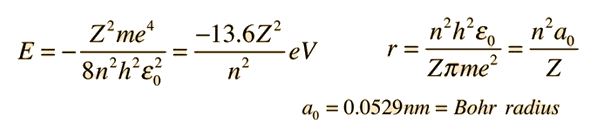# Problem Determine the energy of an electron. Bohrs model fun!

Determine the energy of an electron in the given level of each of the following ions. Use 'e' notation with two decimal places. For example, -2.34e-22.

Hint: Each of the ions has a different number of protons, but each has one electron, so the Bohr model applies to each.
Ions:
Li2+
F8+
Level:
Li^(2+) = 2
F^(8+) = 6

Find Enegery of
Li^(2+) and F^(8+);

Our professor doesn't know how to get it for some reason, he said he might just take it off the hw, but it doesn't seem that hard but maybe we are using hte wrong formula.

In his notes this is the only thing close to what i think it would be:
Oscillating molecules that emit the radiation only have discrete energies
E_n = nhf
f = frequencey
h = planks constant;
n = quantum number
E_n = energy of quantum state n of molecule

for Li^(2+), i'm trying:
Li (Z = 3)
E_n = (3)(6.62E-35Js)(f)
problem is, we don't know the frequency!
any ideas on what other formulas i could use? thanks!

He told us, take Z multiply it by planks constant for ur answer, when we told him it didn't work he said it should have worked hah. SO i dunno whats going on!

## Answers and Replies

Astronuc
Staff Emeritus
Science Advisor
Sorry for the late responce. I'm confused, Z is the atomic number right? The atomic number of Li is 3 right? So using this equation:I let Z = 3, n = 2;
E =-30.6 J;
this isn't right though,is it becuase the atomic number of Li2+ is not 3?
The number i should be getting should be pretty small if it says:
Use 'e' notation with two decimal places. For example, -2.34e-22.

If i have to apply the more complicated form of the formula, i'm confused on what the value of Eo is and what e stands for, could you explain it alittle more? I know h is planks constant, Z is the atomic number, would it still be 3 in this case? m is the mass (6amu), n is the orbit number (n =2), is e the charge of an electron? Thanks for the responce!

Last edited:
nrqed
Science Advisor
Homework Helper
Gold Member
mr_coffee said:
Sorry for the late responce. I'm confused, Z is the atomic number right? The atomic number of Li is 3 right? So using this equation:I let Z = 3, n = 2;
E =-30.6 J;
This is the way to go, except that your answer is in electronvolts not in Joules!

The unit "electron-volt" is very confusing because of the symbol "eV". But in the end it is just a unit of energy, $1 eV = 1.602 \times 10^{-19} J$.

If they expect a small answer, it's probably because they want it in Joules, so you will have to multiply your -30.6 by 1.602 x 10^(-19).
this isn't right though,is it becuase the atomic number of Li2+ is not 3?
The number i should be getting should be pretty small if it says:
Use 'e' notation with two decimal places. For example, -2.34e-22.

They seem to use small "e" for what people usually call "E" (that is, 1e-3 for example = $1 \times 10^{-3}$). This "e" has nothing to do with the electric charge or the "e" in eV.

If i have to apply the more complicated form of the formula, i'm confused on what the value of Eo is and what e stands for, could you explain it alittle more? I know h is planks constant, Z is the atomic number, would it still be 3 in this case? m is the mass (6amu), n is the orbit number (n =2), is e the charge of an electron? Thanks for the responce!
You did it ok (except for confusing eV with Joules). E_0 is simply -13.6 eV. What does "e" stand for? In the formula it is the elementary charge, in "eV", it is just part of the symbol of this unit of energy. In 3e-22 it seems to be a symbol used for scientific notation.

Ahh thank you for the clarification! I got both of them correct! they are both the same answer as well, interesting! thanks again guys!
-4.902e-18J